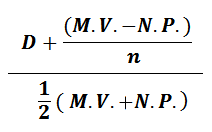222

## \$show=home

### Online Accounting Course  Achieve one of the highest distinctions in the accounting management profession: Become a Online Accountant. As an Online Accountant, you will have the knowledge and the skills to thrive in the competitive accounting industry. The Online Accounting course gives you expertise and real-world skills in accounting analysis. Join more than 100,000 professionals who have been recognized globally for their commitment to ethics and professionalism.## Latest \$type=blogging\$count=10\$author=hide\$comment=hide\$label=hide\$show=home\$date=hide

Cost of preference share capital is that part of cost of capital in which we calculate the amount which is payable to preference shareholders in the form of dividend with fixed rate. Even, dividend to preference shareholder is on the desire of board of directors of company and preference shareholder can not pressurize for paying dividend but it doesn’t mean that calculation of cost of pref. share capital is not necessary because, if we don’t pay the dividend to pref. shareholders, it will affect on capability to receive funds from this source.

Cost of pref. share capital’s formula is given below.

Cost of Pref. Share capital (Kp) = amount of preference dividend/ Preference share capital

Kp = D/P

If we have obtained this preference share capital after some adjustments like premium or discount or pay some cost of floatation, at that time, it is our duty to deduct discount and cost of floatation or add premium in par value of pref. share capital.

In adjustment case cost of pref. share capital will change and we can calculate it with following way:-

Kp = D/ NP

D = Annual pref. dividend,

NP = Net proceed = Par value of Pref. share capital – discount – cost of floatation

Or NP = Par value of pref. share capital + Premium

There will no adjustment of tax rates because, dividend on pref. share capital is payable on net profit after tax adjustment, so need not to do adjustment of tax for comparing it with cost of debt or cost of equity share capital .

Some, time we issue redeemable preference shares whose amount is payable after some time.

At the time of maturity, we need to calculate cost of pref. share capital with following formula

Cost of redeemable pref. share capital =

D = Annual dividend

MV = Maturity value of pref. shares

NP = Net proceeds of pref. shares

N= number of years

This formula is little different from cost of non redeemable pref. share capital because, we have to add, the benefit which we have given to pref. share capital at the time of maturity.

Suppose, we have to pay Rs. 10, 00,000 but at the time of issue of pref. share, we had paid Rs. 2 per issue of pref. share. So, net proceed is Rs. 9,80,000 but if this amount is payable after 10 years at 10% premium, this will also benefit to pref. share capital and total cost of pref. share capital will increase. Rate of dividend is 10%.

Cost of pref. share capital

= D + (MV – NP )/n / ½(MV +NP) X 100

= 100,000 + ( 10,50,000- 9,80,000 )/ ½ ( 10,50,000 + 9,80,000) x 100

= 100,000 + 7,000/ 10, 15,000 X 100

= 10.54%

If we compare it with simple cost of pref. share capital with following way
Kp = D/P X 100 = 100000 / 10, 00,000 X 100 = 10% it is same as dividend rate but Kpr is more than Kp. So, Kpr will give you correct result.: 3
1.X limited issue 1000 debenture or rs 100 each at par interest rate is 8%tax rate is 50% calculate cost of debt capital

2.Np=1000×100=100000
T=50%=.5
I=100000/8%=800
KD=I(1-t)/Np, 800(1-.5)/100000
800(.5)/100000
400/100000=0.04×100=4%

3.A company’s preference share is trading at BSE at Rs. 110. The preference share is a redeemable share and the Company will redeem them after 15 years at a premium of 5% - that is, it will be redeemed after 15 years at Rs. 105; and it is 10% Preference Share – the company will pay a dividend of Rs. 10 every year. You are required to determine the cost of preference shares to the Company.

In comment, you can give your feedback, reviews, ideas for improving content or ask question relating to written content.

Name

ltr
item
Accounting Education: Cost of Preference Share Capital
Cost of Preference Share Capital
http://4.bp.blogspot.com/-77b9sbB1SK4/TzEaJ1UsZAI/AAAAAAAAHS8/k36OmBTbK_M/s1600/123456.PNG
http://4.bp.blogspot.com/-77b9sbB1SK4/TzEaJ1UsZAI/AAAAAAAAHS8/k36OmBTbK_M/s72-c/123456.PNG
Accounting Education
https://www.svtuition.org/2010/04/cost-of-pref-share-capital.html
https://www.svtuition.org/
https://www.svtuition.org/
https://www.svtuition.org/2010/04/cost-of-pref-share-capital.html
true
2410664366776677676
UTF-8